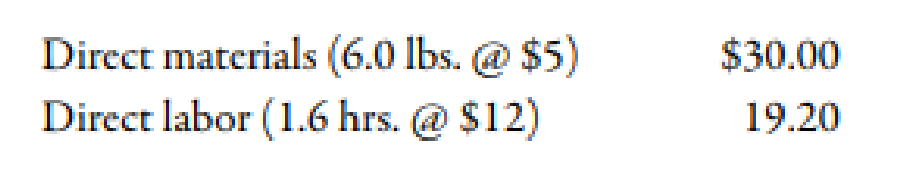Chapter 10, Problem 71P### Managerial Accounting: The Corners...

7th Edition
Maryanne M. Mowen + 2 others
ISBN: 9781337115773

#### Solutions

Chapter
Section### Managerial Accounting: The Corners...

7th Edition
Maryanne M. Mowen + 2 others
ISBN: 9781337115773
Textbook Problem
59 views

# The Lubbock plant of Morril’s Small Motor Division produces a major subassembly for a 6.0 horsepower motor for lawnmowers. The plant uses a standard costing system for production costing and control. The standard cost sheet for the subassembly follows:During the year, the Lubbock plant had the following actual production activity: a. Production of subassemblies totaled 50,000 units. b. A total of 260,000 pounds of raw materials was purchased at $4.70 per pound. c. There were 60,000 pounds of raw materials in beginning inventory (carried at$5 per lb.) There was no ending inventory. d. The company used 82,000 direct labor hours at a total cost of $1,066,000. The Lubbock plant’s practical activity is 60,000 units per year. Standard overhead rates are computed based on practical activity measured in standard direct labor hours.Required: 1. CONCEPTUAL CONNECTION Compute the materials price and usage variances. Of the two materials variances, which is viewed as the more controllable? To whom would you assign responsibility for the usage variance in this case? Explain. 2. CONCEPTUAL CONNECTION Compute the labor rate and efficiency variances. Who is usually responsible for the labor efficiency variance? What are some possible causes for this variance? 3. CONCEPTUAL CONNECTION Assume that the purchasing agent for the small motors plant purchased a lower-quality raw material from a new supplier. Would you recommend that the plant continue to use this cheaper raw material? If so, what standards would likely need revision to reflect this decision? Assume that the end products quality is not significantly affected. 4. Prepare all possible journal entries. 1. To determine Calculate the value of material price variance and material usage variance. Identify which of the material variance is most controllable. Also, identify to whom the responsibility of usage variance is given and give reason. Explanation Variance: The amount obtained when actual cost is deducted from budgeted cost is known as variance. Variance is calculated to find whether the cost is over applied or under applied. Use the following formula to calculate material price variance: Material Price Variance=(Actual PriceStandard Price)×Actual Quantity Substitute$4.70 for actual price, $5.00 for standard price and 260,000 for actual quantity in the above formula. Material Price Variance=($4.70$5.00)×260,000=$78,000(F)

Therefore, the material price variance is $78,000(F). Use the following formula to calculate material usage variance: Material Usage Variance=(Actual QuantityStandard Quantity1)×Standard Price Substitute 320,000 units for actual quantity, 300,000 units for standard quantity and$5 for standard price in the above formula

2.

To determine

Calculate the value of labor rate variance and labor efficiency variance. Identify the person who is responsible for the labor efficiency variance. Also, explain the causes of this variance.

3.

To determine

Identify whether an individual would recommend using the cheaper raw material. If, yes then provide standards that should be used in the decision making.

4.

To determine

Pass necessary journal entries.

### Still sussing out bartleby?

Check out a sample textbook solution.

See a sample solution

#### The Solution to Your Study Problems

Bartleby provides explanations to thousands of textbook problems written by our experts, many with advanced degrees!

Get Started